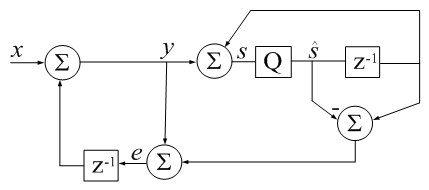# Accumulator Error Feedback

(Difference between revisions)
 Revision as of 20:14, 24 September 2017 (edit)← Previous diff Revision as of 20:17, 24 September 2017 (edit) (undo)Next diff → Line 23: Line 23: % fprintf('sum1 = %18.16e\nsum2 = %18.16e\n', sum1, sum2); % fprintf('sum1 = %18.16e\nsum2 = %18.16e\n', sum1, sum2); - s_hat=0; y=0; e=0; + s_hat=0; e=0; for i=1:numel(x) for i=1:numel(x) s_hat_old = s_hat; s_hat_old = s_hat;

## Revision as of 20:17, 24 September 2017CSUM() in Digital Signal Processing terms: z-1 is a unit delay, Q is a floating-point quantizer to 64 bits, qi represents error due to quantization (additive by definition).
-Jon Dattorro
```function s_hat=csum(x)
% CSUM Sum of elements using a compensated summation algorithm.
%
% For large vectors, the native sum command in Matlab does
% not appear to use a compensated summation algorithm which
% can cause significant roundoff errors.
%
% This code implements a variant of Kahan's compensated
% summation algorithm which often takes about twice as long,
% but produces more accurate sums when the number of
% elements is large. -David Gleich
%
%
% Matlab example:
%   v=rand(1e7,1);
%   sum1 = sum(v);
%   sum2 = csum(v);
%   fprintf('sum1 = %18.16e\nsum2 = %18.16e\n', sum1, sum2);

s_hat=0; e=0;
for i=1:numel(x)
s_hat_old = s_hat;
y = x(i) + e;
s_hat = s_hat_old + y;
e = (s_hat_old - s_hat) + y;  %calculate difference first (Higham)
end
```